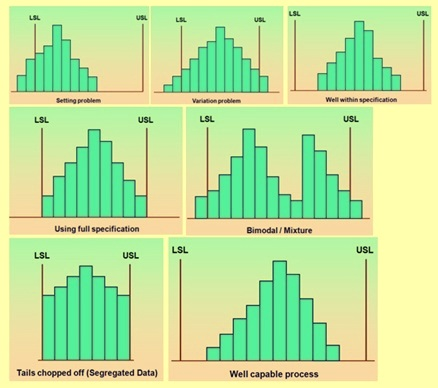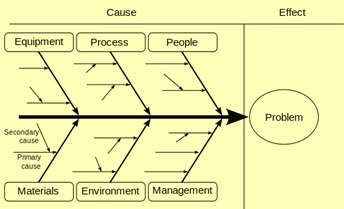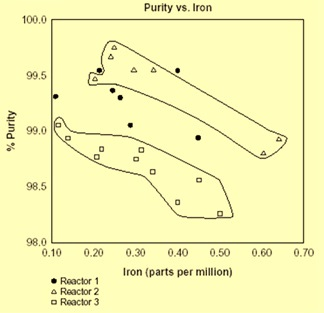Sep20

# Statistical Quality Control

Statistical Quality Control

Statistical quality control (SQC) is the term used to describe the set of statistical tools deployed for evaluating the organizational quality by the quality professionals. Statistical quality control can be divided into following three broad categories.

• Descriptive statistics – These are the statistics used to describe certain quality characteristics such as the central tendency and variability of the observed data. It also describes the relationship. Descriptive statistics include statistics such as the mean, standard deviation, the range, and a measure of the distribution of data.
• Statistical process control (SPC) – It consists of statistical tools that involve inspecting a random sample of the output from a process and deciding whether the process is producing products with characteristics that fall within a predetermined range. SPC answers the question whether the process is functioning properly or not. These tools are very important for a process since they help in identifying and catching a quality problem during the production process.
• Acceptance sampling – It helps in evaluating whether there is problem with quality and whether desirable quality is being achieved for a batch of product. Accepting sampling consists of the process of randomly inspecting a sample of goods and deciding whether to accept the entire lot based on the results. This sampling decides whether a batch of goods is to be accepted or rejected.

There are seven basic tools employed for SQC. The seven basic tools of quality is a designation given to a fixed set of graphical techniques identified as being most helpful in trouble shooting issues related to quality. They are called basic because they are suitable for people with little formal training in statistics and because they can be used to solve the vast majority of quality related issues. These seven basic tools are described below.

Check sheets

Check sheets are simple data gathering devices. Check sheets are used to collect data effectively and efficiently. They prepare data for further analysis. A check sheet is in the form of a designed format used to collect data in a systematic manner and in real time at the location where the data is generated. The data it captures can be quantitative or qualitative. When the information is quantitative, the check sheet is sometimes called a tally sheet. The defining characteristic of a check sheet is that data are recorded by making marks (checks) on it. A typical check sheet is divided into regions, and marks made in different regions have different significance. Data are read by observing the location and number of marks on the sheet. Various types of check sheets are

(i)                Process distribution check sheet,

(ii)              Defect cause check sheet

(iii)             Defect location check sheet

(iv)             Defect cause-wise check sheet.

Histogram

A histogram is a graphical representation (bar chart) of the distribution of data. It is an estimate of the probability distribution of a continuous variable and was first introduced by Karl Pearson. A histogram is a representation of tabulated frequencies, shown as adjacent rectangles, erected over discrete intervals (bins), with an area equal to the frequency of the observations in the interval. The height of a rectangle is also equal to the frequency density of the interval, i.e., the frequency divided by the width of the interval. The total area of the histogram is equal to the number of data.

A histogram may also be normalized displaying relative frequencies. It then shows the proportion of cases that fall into each of several categories, with the total area equaling one. The categories are usually specified as consecutive, non-overlapping intervals of a variable. The categories (intervals) must be adjacent, and often are chosen to be of the same size. The rectangles of a histogram are drawn so that they touch each other to indicate that the original variable is continuous. Histograms are used to plot the density of data, and often for density estimation which is the estimating the probability density function of the underlying variable. The total area of a histogram used for probability density is always normalized to one.

Histograms are used to understand the variation pattern in a measured characteristic with a reference to location and spread. They give an idea about the setting of a process and its variability. Histograms indicate the ability of the process to meet the requirements as well as the extent of the non conformance of the process. Different patterns of histogram are shown in Fig 1.Fig 1 Different patterns of histogram

Pareto analysis

Ideally one wants to focus his attention on fixing the most important problem. Pareto Analysis is a simple and formal technique that helps to identify the top portion of causes that are to be addressed to resolve the majority of problems. It is a decision-making technique that statistically separates a limited number of input factors as having the greatest impact on an outcome, either desirable or undesirable. In its simplest terms, pareto analysis typically shows that a disproportionate improvement can be achieved by ranking various causes of a problem and by concentrating on those solutions or items with the largest impact. The basic premise is that not all inputs have the same or even proportional impact on a given output.

Pareto Analysis is also referred as the ‘80/20 rule’. Under this rule, it is assumed that 20 percent of causes when addressed to, generate 80 percent of results. Pareto analysis tool is used to find the 20 percent of those causes which when addressed will resolve 80 percent of the problems. This ratio is merely a convenient rule of thumb and is not to be considered as law of nature.

Pareto analysis is a creative way of looking at causes of problems because it helps stimulate thinking and organize thoughts. However, it can be limited by its exclusion of possibly important problems which may be small initially, but which grow with time. It should be combined with other analytical tools such as failure mode and effects analysis and fault tree analysis for example.

The application of the Pareto analysis in risk management allows management to focus on those risks that have the most impact. A pareto analysis chart is shown in Fig 2.Fig 2 Pareto analysis chart

Control chart

A control chart is a graph that shows whether a sample of data falls within the common or normal range of variation. A control chart has upper and lower control limits (UCL and LCL) which separate common from assignable causes of variation. The UCL is the maximum acceptable variation from the mean for a process that is in a state of control while the LCL is the minimum acceptable variation from the mean for a process that is in a state of control. The common range of variation is defined by the use of control chart limits. Control limits are calculated from the process output data and they are not specification limits. A process is out of control when a plot of data reveals that one or more samples falls outside the preset control limits.

Control charts are one of the most commonly used tools. They can be used to measure any characteristics of a product. These characteristics can be divided into two groups namely variables and attributes. A control chart for a variable is used to monitor that can be measured and has a continuum of values. On the other hand, a control chart for attributes is used to monitor characteristics that have discreet values and can be counted. Often attributes are evaluated with a simple yes or no decision.

Control chart gives signal before the process starts deteriorating. It aids the process to perform consistently and predictably. It gives a good indication of whether problems are due to operation faults or system faults. Typical control chats are given in Fig 3.Fig 3 Typical control charts

Various types of control charts are as follows.

• Mean or x-bar chart – It is control chart is used to monitor changes in the mean value or shift in the central tendency of a process.
• Range (R) chart – This chart monitors changes in the dispersion or variability of the process.
• p-chart – This is a control chart which is used to measure the proportion that is defective in a sample. The centre line in this chart is computed as the average proportion defective in the population p. A p-chart is used when both the total size and the number of defects can be computed.
• c-chart – c-chart is used to monitor the number of defects per unit. A c-chart is used when we can compute only the number of defects but cannot compute the proportion that is defective

Cause and effect analysis diagram

When one is able to relate different causes to the effect, namely the quality characteristics, then he can use this logical thinking of cause and effect for further investigations to improve and control quality. This type of linking is done through cause and effect diagrams.

Cause and effect analysis was devised by Professor Kaoru Ishikawa, a pioneer of quality management, in 1968. Cause and effect analysis diagram is also known as Ishikawa, Herringbone or Fishbone diagram (since a completed diagram can look like the skeleton of a fish). Cause and effect analysis diagram technique combines brainstorming with a type of mind map. It pushes one to consider all possible causes of a problem, rather than just the ones that are most obvious.

Cause and effect analysis diagrams are casual diagrams which show the causes of a specific event. Common uses of these diagrams are product design and quality defect prevention, to identify potential factors causing an overall effect. Each cause or reason for imperfection is a source of variation. Causes are normally grouped into six major categories to identify these sources of variation. These six categories are as follows.

• People – It include any person involved with the process.
• Methods – Methods include how the process is performed and the specific requirements for doing it, such as policies, procedures, rules, regulations and laws etc.
• Machines – Under machines comes equipment, computers and tools etc. which are required to accomplish the job.
• Materials – Materials include raw materials, consumables, spare parts, pens and paper, etc. used to produce the final product.
• Measurements – These are the data generated from the process that are used to evaluate its quality.
• Environment – These are the conditions, such as location, time, temperature, and culture in which the process operates.

A cause and effect diagram is shown in Fig.4.Fig4  Cause and effect diagram

Stratification

Stratification is a technique used in combination with other data analysis tools. When data from a variety of sources or categories have been lumped together, the meaning of the data can be impossible to see. The technique separates the data so that the pattern can be seen. Stratification can be used in the cases (i) before collecting the data (ii) when data come from several sources or conditions such as shifts, days of week, suppliers, materials, products, departments, equipment, or population groups and (iii) when data analysis may require separating different sources or conditions. Stratification procedure consists of the following three steps.

• Before collecting data, one has to consider which information about the sources of the data might have effect on the results. During data collection data related to this information are to be collected as well.
• When plotting or graphing the collected data on a scatter diagram, control chart, histogram or other analysis tool, different marks or colours need to be used to distinguish from data from various sources. Data that are distinguished in this way are said to be ‘stratified’.
• The subsets of stratified data are analyzed separately.

A n example of data stratification is given in Fig. 5 on a scatter diagram.Fig 5 Example of data stratification

Scatter diagram

The scatter diagram graphs pairs of numerical data, with one variable on each axis, to look or relationship between them. If the variables are correlated, the points will fall along a line or curve. A scatter diagram is a type of mathematical diagram using Cartesian coordinates to display values for two variables for a set of data. The data is displayed as a collection of points, each having the value of one variable determining the position on the horizontal axis and the value of the other variable determining the position on the vertical axis. A scatter diagram is used when a variable exists that is below the control of the operator. If a parameter exists that is systematically incremented and/or decremented by the other, it is called the control parameter or independent variable and is customarily plotted along the horizontal axis. The measured or dependent variable is customarily plotted along the vertical axis. If no dependent variable exists, either type of variable can be plotted on either axis or a scatter diagram will illustrate only the degree of correlation (not causation) between two variables. A typical scatter diagram is shown in Fig.6.Fig 6 Scatter diagram

Terms used in SQC

The following are some of the terms used in the statistical quality control.

• Mean – It is an important statistic tool that measures the central tendency of a set of data. The mean is computed by simply summing up of all the observations and dividing by the total number of observations.
• Range and standard deviation – This information provides with the variability of the data. It tells how the data is spread out around the mean.  Range is the difference between the largest and the smallest observations in a set of data while standard deviation is a statistics that measures the amount of data dispersion around the mean. Small values of the range and standard deviation mean that the observations are closely clustered around the mean while large values of the range and standard deviation mean that the observations are spread around the mean.
• Distribution of data – It is a measure to determine the quality characteristics. When the distribution of data is symmetric then there are same numbers of observations below and above the mean. This is what is commonly found when only normal variation is present in the data. When a disproportionate number of observations are either above or below the mean, then the data has a skewed distribution.